Using Minimal Polynomial Bases for Model-Based Fault Diagnosis

Vehicular Systems group
Department of Electrical Engineering, Linkoping University
SE-581 83 Linkoping, Sweden

 To download the application in .pdf format (97 k), click here.

Abstract
A brief introduction to the residual generation problem for fault diagnosis in linear systems is given and a solution based on polynomial methods are outlined. Also, a design example, complete with MATLAB code illustrates how the Polynomial Toolbox can be used in the design of residual generators. For more detailed information on the design method, see e.g. (Frisk & Nyberg 1999b, Nyberg & Frisk 1999).

1 Introduction
A model based diagnosis system commonly consists of a residual generator followed by thresholds and some decision logic. The residual generator filters the known signals and generates a signal, the residual, that should be small (ideally 0) in the fault-free case and large when a fault is acting on the system. In Figure 1, it is illustrated how the residual generator is connected to the real system. The figure also shows that not only the control signal u influences the system, but also disturbances d and the faults f that we wish to detect. Both disturbances and faults are here modeled as input signals to the system. In order not to make the residual sensitive to the disturbances d, the disturbances must be decoupled. By using several residuals, or a vector-valued residual, where not only disturbances but also different subset of faults are decoupled, it is possible to achieve isolation. Isolation means distinguishing between different faults and locate the fault component. This is the basic idea of a diagnosis system using the principle of structured residuals (Gertler 1998) or the more general principle of structured hypothesis tests (Nyberg 1999). The set of faults that, along with the disturbances, are decoupled in a residual are called non-monitored faults.Figure 1: A residual generator.

2 Theory
This demonstration shows linear residual generation for linear systems with no model uncertainties. A general linear residual generator can be written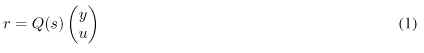i.e. Q(s) is a multi-dimensional transfer-matrix with known signals y(t) and u(t) as inputs and a scalar residual as output. The requirement on the residual generator, i.e. Q(s), is that it is sensitive to monitored faults and not sensitive to disturbances (including non-monitored faults). This section introduces linear residual generation problem and also briefly describes the minimal polynomial basis solution. All derivations are performed in the continuous case but the corresponding results for the time-discrete case can be obtained by substituting s by z and improper by non-causal.

2.1 Linear Residual Generation
The systems studied in this work are assumed to be on the formwhere y(t) is measurements, u(t) is known inputs to the system, d(t) is unknown disturbances including non-monitored faults, and f(t) is the monitored faults. The filter Q(s) in (1) is a residual generator if and only if the transfer function from u and d to r is zero, i.e. limt !1 r(t) = 0 for all d(t) and u(t) when f(t) = 0. To be able to detect faults, it is also required that r(t) <> 0 when f(t) <> 0.

Inserting (2) into (1) givesTo make r(t) = 0 when f(t) = 0, it is required that disturbances and the control signal are decoupled, i.e. for Q(s) to be a residual generator, it must hold thatAlso, it is required that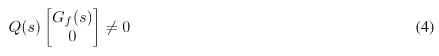with suitable properties, e.g. adequate DC-gain from faults. Thus, the linear residual generation problem is to find a suitable Q(s) that fulfills (3) and (4).

2.2 A Solution based on Minimal Polynomial Bases
Equation (3) implies that Q(s) must belong to the left null-space ofThis null-space is denoted NL(M(s)). The matrix Q(s) thus need to fulfill two requirements: belong to the left null-space of M(s) and give good fault sensitivity properties in the residual. If, in a first step of the design, all Q(s) that fulfills the first requirement is found, then a Q(s) with good fault sensitivity properties can be selected. Thus, in a first step of the design of the residual generator Q(s) we need not consider f or Gf (s). The problem is then to find all rational Q(s) that belong to NL(M(s)). Of special interest are the residual generators with least McMillan degree, i.e. the number of states in a minimal realization. Finding all Q(s) in NL(M(s)) can be done by finding a minimal basis for the rational vector-space NL(M(s)). A minimal basis for a rational vector-space is a polynomial basis (Forney 1975). For now, assume that such a basis can be found and let the base vectors be the rows of a matrix denoted NM(s). How to extract such a basis will be dealt with in Section 2.3. By inspection of (5), it can be realized that the dimension of NL(M(s)) (i.e. the number of rows of NM(s) iswhere m is the number of outputs, i.e. the dimension of y(t), and kd is the number of disturbances, i.e. the dimension of d(t). The last equality holds only if rank Gd(s) = kd, but this should be the normal case. When a polynomial basis NM(s) has been obtained, the second and final step in the residual generator design is to shape fault-to-residual responses as described next. The minimal polynomial basis NM(s) is irreducible and because of this (Kailath 1980), all decoupling residual generators Q(s) can be parameterized aswhere(s) is an arbitrary rational vector of suitable dimensions. This parameterization vector(s) can e.g. be used to shape the fault-to-residual response or simply to select one row in . Since NM(s) is a basis, the parameterization vector(s) have minimal number of elements. The only constraint on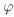(s) if the residual generator Q(s) is to be realizable, it must be chosen such that (6) is proper. This means that the dynamics, i.e. poles, of the residual generator Q(s) can be chosen freely. This also means that the minimal order of a realization of a decoupling filter is determined by the row-degrees of the minimal polynomial basis NM(s).

2.3 Finding the Minimal Polynomial Basis
The problem of finding a minimal polynomial basis to the left null-space of the rational matrix M(s) can be solved by a transformation to a problem of finding a minimal polynomial basis to the left null space of a polynomial matrix. This transformation can be done in several different ways. In this section, two possibilities are demonstrated, where one is used if the model is given on transfer function form and the other if the model is given in state-space form. The motivation for this transformation to a purely polynomial problem, is that there exists well established theory (Kailath 1980) for polynomial matrices. In addition, the generally available software, (The Polynomial Toolbox 2.0 for Matlab 5 1998), contains an extensive set of tools for numerical handling of polynomial matrices.

State-Space Solution
Assume that the system is described in state-space form,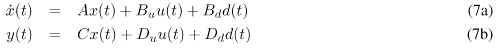Then it is convienient to use the system matrix in state-space form (Rosenbrock 1970) to find the left null-space to M(s). Denote the system matrix Ms(s), describing the system with disturbances as inputs: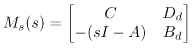Also define the matrix P as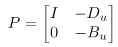The proof of this theorem can be found in (Frisk & Nyberg 1999a). In conclusion, the problem of finding a minimal polynomial basis to a general rational matrix has been transformed into finding a minimal polynomial basis to a specific matrix pencil, in this case the system matrix Ms(s).

Frequency Domain Solution
If the model is given on transfer function form, one way of transforming the rational problem to a polynomial problem is to perform a right MFD on M(s), i.e.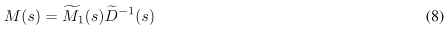One simple example is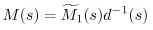3 Design Example: Aircraft Dynamics
This model, taken from (Maciejowski 1989), represents a linearized model of vertical-plane dynamics of an aircraft. This section includes MATLAB code of central operations. The inputs and outputs of the model areThe following Matlab-code defines model equations:

%% Define state-space matrices
>> A =  [0, 0, 1.1320, 0,-1
0,-0.0538,-0.1712, 0,0.0705
0, 0, 0, 1,0
0, 0.0485, 0,-0.8556,-1.0130
0,-0.2909, 0, 1.0532,-0.6859];

>> B = [0,0,0
-0.1200,1,0
0,0,0
4.4190,0,-1.6650
1.5750,0,-0.0732];

>> C = [eye(3) zeros(3,2)];
>> D = zeros(3,3);

%% Form the transfer functions and initialize some constants
>> Gu = ss(A,B,C,D);
>> n = size(A,1);
>> [nmeas,nctrl] = size(Gu);

Suppose the faults of interest are sensor-faults (denoted f1 , f2 , and f3 ), and actuator-faults (denoted f4 , f5 , and f6 ). Also, assume that the faults are modeled with additive fault models. The total model, including fault models then becomes:where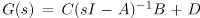Thus, the transfer function from fault vector f to measurement vector y becomes, Gyf (s) = [I3 G(s)]. The design example is to design a residual generator Q(s) that decouples faults in the elevator angle actuator, i.e. f6 is considered a disturbance during the design. The matrix Gd(s) from Equation 2 corresponds to all signals that are to be decoupled, i.e. considered disturbances. In this case, Gd(s) becomes the column in Gyf (s) corresponding to f6 , i.e. the third column in G(s). Matrix Gf(s) corresponds to the monitored faults and therefore Gf(s) becomes the rest of the columns of Gyf (s). The following Matlab-code defines the transferfunctions from faults and disturbances.

>> Gd = Gu(:,3);
>> Gyf = [eye(3,3) Gu];
>> ndist = size(Gd,2);
>> nfault = size(Gyf,2);

The next step in the design is to find NM(s). Before using Theorem 1, the controllability requirement needs to be checked. Matlab gives

>> B_d = B(:,3);
>> D_d = D(:,3);
>> rank(ctrb(A,[B B_d]))
ans =
5

i.e. the controllability requirement is fulfilled. The Polynomial Toolbox macro null (computation of the right null-space of a polynomial matrix) can then be used to compute the required left null-basis with the help of the transpose operator

>> Ms = [C D_d;-(s*eye(n)-A) B_d)];

>> P = [eye(nmeas) -D;zeros(n,nmeas) -B];

>> Nm = null(Ms.’).’*P
Nm =
0.071s 0.054 + s 0.091 0.12 -1 0
15s + 23sˆ2 -6.7 -17 - 0.94s + sˆ2 31 0 0

The second method, the frequency domain approach can also be used. Direct calculations give

>> M = minreal([Gu Gd;eye(nctrl) zeros(nctrl,ndist)]);
>> [N,D] = ss2rmf(M.a, M.b, M.c, M.d);
>> Nm2 = null(N.’).’
Nm2 =
0.071s 0.054 + s 0.091 0.12 -1 0
15s + 23sˆ2 -6.7 -17 - 0.94s + sˆ2 31 0 0

where ss2rmf is a macro of the Polynomial Toolbox that converts a state-space model to a right MFD. As can be seen, the bases are identical. A minimal polynomial basis is not unique, but the algorithm implemented in the toolbox null command is based on a canonical echelon form resulting in identical bases. The above basis has a natural interpretation. It means that the following equations hold in the fault-free case and also for all values of the actuator fault f6 = d.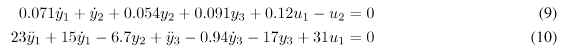The final step in the design is to choose the parametrization vector '(s). In a first design, utilize the first relation (9) and add low-pass dynamics by setting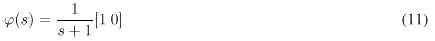Matlab-code to form the residual generator:

>> [Qa,Qb,Qc,Qd] = lmf2ss([1,0]*Nm,s+1);
>> Q = ss(Qa,Qb,Qc,Qd);

To evaluate the design, both decoupling properties and fault sensitivity need to be analyzed. Figure 2 shows the transfer functions from faults to the residual and the decoupling of disturbances and control signals is evaluated by calculating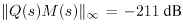It is clear that the decoupling has suceeded, down to machine precision, and that there is a nonzero gain from all faults besides f6 which was to be decoupled. Since the null-space had two basis vectors, we have some freedom in using both these relationships, i.e. (9) and (10), to form the residual generator. By letting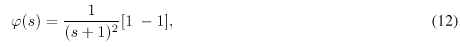i.e. use a linear combination of the two basis vectors and add 2nd order low-pass dynamics to form the residual generator. Here, at least a 2nd order low-pass link was needed since the row-degree of the second basis vector was 2. The residual generator is also normed to have the same DC-gain as the first design to be able to do some comparison. Matlab-code to form the residualgenerator:

>> [Q2a,Q2b,Q2c,Q2d] = lmf2ss([1,-1]*Nm,(s+1)ˆ2);
>> Q2 = ss(Q2a,Q2b,Q2c,Q2d);

% Normalize to equal DC-gain of Q(s) and Q2(s)
>> Q2 = svd(evalfr(minreal(Q),0))/svd(evalfr(minreal(Q2),0))*Q2;

Evaluation of decoupling and fault sensitivity properties of this second design is shown in Figures 3 where fault sensitivity is shown. calculating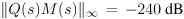shows that the decoupling, also here has succeded down to machine precision. This second design shows how the design freedom available in '(s) can be used to shape important transfer functions and still keep the important decoupling property.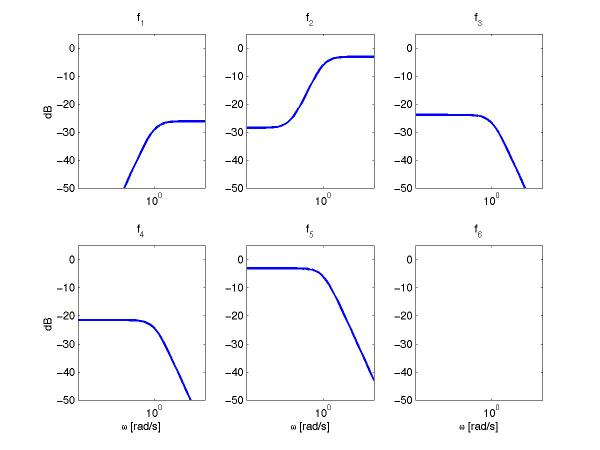Figure 2: Transfer functions from the 6 faults to the residual. Note that fault 6 is decoupled, i.e. gain 0 from f6 to r.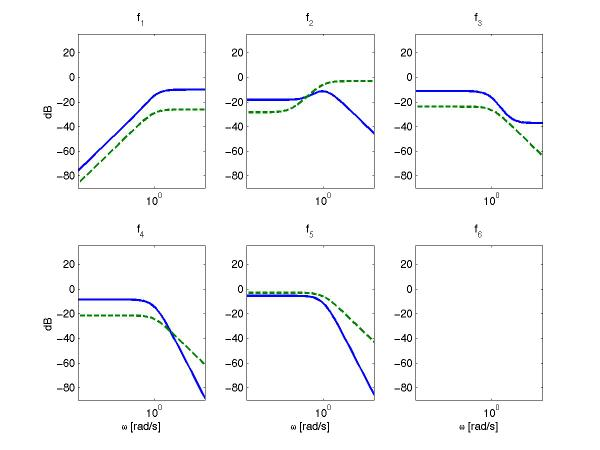Figure 3: Transfer functions from the 6 faults to the residual. Note that fault 6 is decoupled, i.e. gain 0 from f6 to r. The dashed lines corresponds to the results of the first design with '(s) given by (11). The solid lines corresponds to the second design where '(s) given by (12).

References

Forney, G. (1975). Minimal bases of rational vector spaces, with applications to multivariable linear systems, SIAM J. Control 13(3): 493–520.

Frisk, E. & Nyberg, M. (1999a). A description of the minimal polynomial basis approach to linear residual generation, Technical Report LiTH-R-2088, ISY, Linkoping, Sweden.

Frisk, E. &Nyberg, M. (1999b). Using minimal polynomial bases for fault diagnosis, European Control Conference, Karlsruhe, Germany.

Gertler, J. (1998). Fault Detection and Diagnosis in Engineering Systems, Marcel Dekker.

Kailath, T. (1980). Linear Systems, Prentice-Hall.

Maciejowski, J. (1989). Multivariable Feedback Design, Addison Wesley.

Nyberg, M. (1999). Framework and method for model based diagnosis with application to an automotive engine, European Control Conference.

Nyberg, M. & Frisk, E. (1999). A minimal polynomial basis solution to residual generation for fault diagnosis in linear systems, Vol. P, IFAC, Beijing, China, pp. 61–66.

Patton, R., Frank, P. & Clark, R. (eds) (1989). Fault diagnosis in Dynamic systems, Systems and Control Engineering, Prentice Hall.

Rosenbrock, H. (1970). State-Space and Multivariable Theory, Wiley, New York.

The Polynomial Toolbox 2.0 for Matlab 5 (1998). Polyx, Czech Republic. URL: http://www.polyx.com.